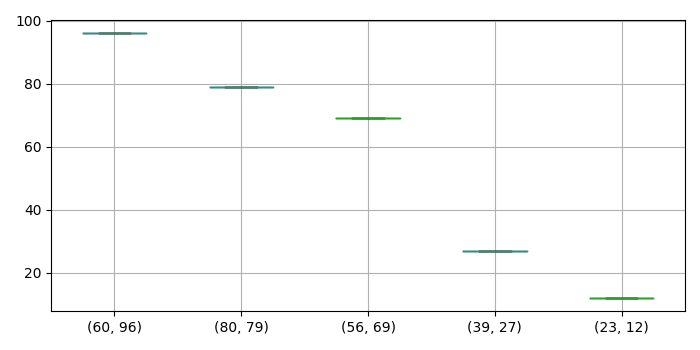# How to sort a boxplot by the median values in Pandas?

To sort a boxplot by the median values in Pandas, we can take the following steps

## Steps

• Set the figure size and adjust the padding between and around the subplots.

• Create a Pandas dataframe of two-dimensional, size-mutable, potentially heterogeneous tabular data, with three columns.

• Group the dataframe elements by marks and dob.

• Find the median of the dataframe.

• Get the sorted values of the median.

• Create a box plot from the DataFrame columns.

• To display the figure, use Show() method.

## Example

import pandas as pd
import matplotlib.pyplot as plt

plt.rcParams["figure.figsize"] = [7.00, 3.50]
plt.rcParams["figure.autolayout"] = True

df = pd.DataFrame([
[23, 'James', 12],
[39, 'Jimmy', 27],
[56, 'Jack', 69],
[60, 'Tom', 96],
[80, 'Tim', 79]
], columns=['marks', 'names', 'dob'])

g = df.groupby(["marks", "dob"])

df = pd.DataFrame({col: val['dob'] for col, val in g})

median = df.median()
median.sort_values(ascending=False, inplace=True)

df = df[median.index]
df.boxplot()

plt.show()

## Output

It will produce the following output −Updated on: 19-Oct-2021

880 Views